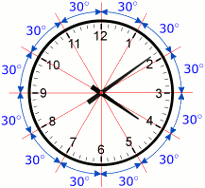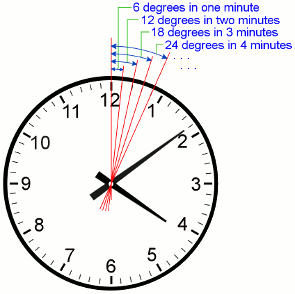# Logical Reasonning: Clock ProblemsLast Updated on Nov 1, 2020

Clock problems are one of the frequently asked questions in most of the competitive exam under Logical Reasoning. To solve these problems, it is always better to understand some of the basic principles and the types of problems that get asked. In this post, we will discuss simple tricks and some simple formulas for solving clock problems.

#### Basics Of Clocks

An analog clock is a circle and the basic principle starts with the fact that there are 360 degrees of angular measurement in a circle.

Next, we had to determine the measure in degrees between the increment of 1 hour. Since there are 12 hours on a standard clock face we divided the total 360-degree measurement by 12 to get 30. 360/12=30. This tells us that the space between any 2 hours is 30 degrees.As the minute hand takes a complete round in one hour it covers 360° in 60 min.
In 1 minute it covers 360/60 = 6°/ minute.Also, as the hour hand covers just one part out of the given 12 parts in one hour. This implies it covers 30° in 60 min. i.e. ½° per minute.
This implies that the relative speed of the minute hand is 6 – ½ = 5 ½ degrees.

• Every hour, both the hands coincide once. But in 12 hours, they will coincide 11 times and this happens due to only one such incident between 12 and 1’o clock
• The hands are in the same straight line when they are coincident or opposite to each other
• When the two hands are at a right angle, they are 15-minute spaces apart. In one hour, they will form two right angles and in 12 hours there are only 22 right angles. It happens due to right angles formed by the minute and hour hand at 3’o clock and 9’o clock
• When the hands are in opposite directions, they are 30-minute spaces apart
• If both the hour hand and minute hand move at their normal speeds, then both the hands meet after 65(5/11) minutes

#### Types of Clock Problems

###### Type 1: Finding the time when the angle between the two hands is given.

Example: At what time between 2’O clock and 3’O clock the hands of the clock were opposite to each other.
1. 34( 6/11 ) past 2’Oclock
2. 43( 7/11 ) past 2’Oclock
3. 56( 8/11 ) past 2’Oclock
4. 64(9/11past 2’Oclock

Solution:

At 2’O clock, the minute hand will be at 12 as shown belowThe minute’s hand to coincide with the hour hand it should trace at first 10 minute spaces and then the hands of the clocks to be opposite to each other minute hand should trace 30-minute spaces i.e. totally it should gain 10+30=40 minute spaces to be opposite to that of the hour hand
We know that,
Minute hand gains 55-minute spaces over hour hand in 1 hour
Therefore, Minute hand gain 40-minute spaces over hour hand in 40 × (60/55) = 43(7/11)
Hence the hand of the clock will minute be opposite to each at 43( 7/11 ) past 2’Oclock
Therefore, the Correct option is ‘2’.

###### Type 2: Finding the angle between the 2 hands at a given time.

Example: What is the angle between the hands of the clock at 7:20

Solution:

At 7 o’ clock, the hour hand is at 210 degrees from the vertical.
In 20 minutes,
Hour hand = 210 + 20*(0.5) = 210 + 10 = 220 {The hour hand moves at 0.5 degree per minute}
Minute hand = 20*(6) = 120 {The minute hand moves at 6 degree per minute }
Difference or angle between the hands = 220 – 120 = 100 degrees

###### Type 3: Questions on clocks gaining/losing time.

Such sort of problems arises when a clock runs faster or slower than expected pace. When solving these problems it is best to keep track of the correct clock.

Example: A watch gains 5 seconds in 3 minutes and was set right at 8 AM. What time will it show at 10 PM on the same day?

Solution:

The watch gains 5 seconds in 3 minutes = 100 seconds in 1 hour.
From 8 AM to 10 PM on the same day, time passed is 14 hours.
In 14 hours, the watch would have gained 1400 seconds or 23 minutes 20 seconds.
So, when the correct time is 10 PM, the watch would show 10: 23: 20 PM

### Summary

In this post, we have explained Basics of a Clock, Facts of Clocks and Types of Clocks with a simple example. For more related posts you can check the link below.
Seating Arrangement Problems

If you liked our post, let us know in the comment section below.